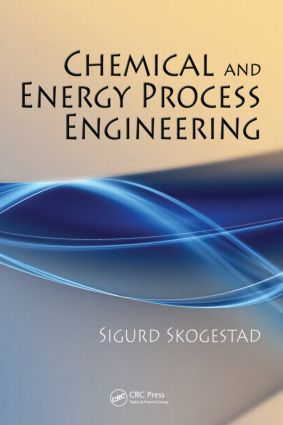# Chemical and Energy Process Engineering

## 1st Edition

CRC Press

440 pages | 145 B/W Illus.

##### Purchasing Options:\$ = USD
Hardback: 9781420087550
pub: 2008-08-27
SAVE ~\$26.00
\$130.00
\$104.00
x
eBook (VitalSource) : 9780429144493
pub: 2008-08-27
from \$65.00

FREE Standard Shipping!

### Description

Emphasizing basic mass and energy balance principles, Chemical and Energy Process Engineering prepares the next generation of process engineers through an exemplary survey of energy process engineering, basic thermodynamics, and the analysis of energy efficiency. By emphasizing the laws of thermodynamics and the law of mass/matter conservation, the author builds a strong foundation for performing industrial process engineering calculations. The book’s systematic treatment applies these core principles on a macro-level scale, allowing for more manageable calculations.

The development of new processes is demanding and exciting. The instruction within these pages enables engineers to understand and analyze existing processes and primes them for participation in the development of new ones.

Notation, concepts and numbers

Notation

Always check the units!

Some conversion factors

Some important numbers

Some important concepts

Unit operations

Batch and continuous process

A little about economy

Some fun and useful energy exercises

Global energy consumption

Derivation of balance equations

The balance principle

The balance equation

Mass balances without accumulation

Recycle

Systematic formulation and solution of mass balances

Use of spreadsheet program

Examples of recycle without reaction

Flash calculations

Summary: Procedure for deriving balance equations

Degrees of freedom and solvability

Simulation versus design

Summary

Mass balances with reaction

Introduction

The component balance

Conversion and extent of reaction

Selectivity and yield

Reaction and recycle

Atomic balances

Independent reactions and matrix formulation

Reaction with chemical equilibrium

Summary

The energy balance

The general energy balance (open system)

Energy forms

Work forms

Alternative formulations of the energy balance

Calculation of enthalpy

Energy balance for mixing processes

Valve: Isenthalpic pressure relief

Real fluids: Thermodynamic state diagrams

Energy balance with chemical reaction

Energy balance with kinetic and potential energy

Summary of energy balance

Heat exchange

Introduction

Calculation (design) of heat exchangers

Simulation of heat exchangers

Compression and expansion

Introduction

Compression (increase of pressure)

Expansion in turbine

Reversible shaft work

Reversible shaft work for ideal gas

Actual work and examples

Pump work

Compression and expansion of real gases

Entropy and equilibrium

The laws of thermodynamics

Calculation of entropy

Equilibrium

Introduction to vapor/liquid equilibrium

Flash calculations

Work from heat

Thermodynamics

Heat engine and the first law

Heat engine and the second law

Reverse heat engine: Refrigeration and heat pump

Efficiency

Ideal work and exergy

Gas power plant

Summary

Mechanical energy balance

The "regular" energy balance

Mechanical energy

Reversible shaft work and friction

The mechanical energy balance

Compressible flow in pipe (gases)

A remark on friction

Summary

Chemical reaction engineering

Reaction kinetics

Reactor calculations and reactor design

Process dynamics

Introduction

Modeling: Dynamic balances

Dynamic analysis and time response

Linearization

Dynamic simulation with examples

Process control

Summary

Appendices

Some thermodynamics and physical chemistry

More thermodynamics: Entropy and equilibrium

Differential balances: Examples

Summary of the whole book